# KSEEB Solutions for Class 10 Maths Chapter 1 Arithmetic Progressions Ex 1.1

Students can Download Class 10 Maths Chapter 1 Arithmetic Progressions Ex 1.1 Questions and Answers, Notes Pdf, KSEEB Solutions for Class 10 Maths helps you to revise the complete Karnataka State Board Syllabus and to clear all their doubts, score well in final exams.

## Karnataka State Syllabus Class 10 Maths Chapter 1 Arithmetic Progressions Ex 1.1

Question 1.
In which of the following situation, does the list of numbers involved make an arithmetic progression, and why?
(i) The taxi fare after each km when the fare is ₹15 for the first km and ₹8 for each additional km.
i) Rent for            Rent for            Rent for
1st km                  2nd km             3rd km
Rs. 15                     Rs. 8                 Rs. 8
d = a2 – a1 = 8 – 15 = -7
d = a3 – a2 = 8 – 8 = 0
Here ’d’ is not constant.
Hence it does not form an AP.

(ii) The amount of air present in a cylinder when a vacuum pump removes $$\frac{1}{4}$$ of the air remaining in the cylinder at a time.
The amount of air present in the cylinder = a1 = x units.
Amount of air present in the cylinder after the first-time removalAmount of air present in the cylinder after second-time removal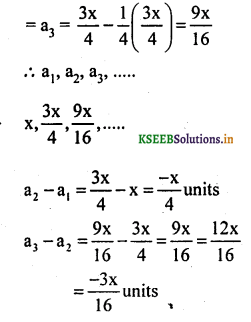a2 – a1 = a3 – a2
∴ a, a2, a3 does not form an A.P

(iii) The cost of digging a well after every metre of digging, when it costs ₹ 150 for the first metre and rises by ₹ 50 for each subsequent metre.
Cost of digging the well for first metre = a1 = ₹ 150
Cost of digging the well for second metre = a2 = 150 + 50 = ₹ 200
Cost of digging the well for third metre = a3 = 200 + 50 = ₹ 250
∴ a1, a2, a3,…….
150, 200, 250, ………
a2 – a1 = a3 – a2 = a4 – a3
200 -150 = 250 – 200 = 300 – 250
50 = 50 = 50
So this list of numbers form an A.P. therefore the common difference, d = ₹ 50.

(iv) The amount of money in the account every year, when ₹ 10000 is deposited at compound interest at 8% per annum.
We know that amount of present value p at r% compound interest after ‘n’ years.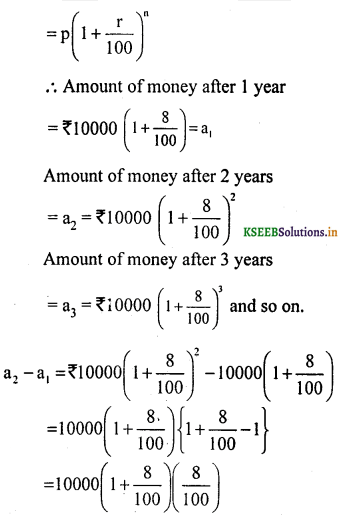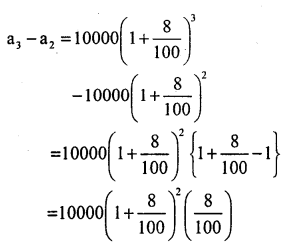a2 – a1 ≠ a3 – a2
∴ a1, a2, a3 does not from an A.P

Question 2.
Write first four terms of the AP, when the first term a and the common difference d are given as follows:
(i) a = 10, d = 10
(ii) a = -2, d = 0
(iii) a = 4, d = -3
(iv) a = -1, d = $$\frac{1}{2}$$
(v) a = -1.25, d = -0.25
i) a = 10, d = 10
First four terms are,
a, a + d, a + 2d, a + 3d
10, 10 + 10, 10 + 20, 10 + 30
10. 20, 30, 40.

ii) a = -2, d = 0
First four terms are,
a, a + d, a + 2d, a + 3d
-2. -2 + 0, -2 + 0, -2 + 0
-2. -2. -2, -2

iii) a = 4, d = -3
First four terms are,
a, a + d, a + 2d, a + 3d
4, 4 – 3, 4 – 2 × 3, 4 – 3 ×3
4, 1, -2, -5

iv) a = – 1 , d = $$\frac{1}{2}$$
Four terms of an A.P are a, a + d, a + 2d, a + 3d.
First term = a = – 1
Second term = a + d∴ Four terips of an AP are
$$-1, \frac{-1}{2}, 0, \frac{1}{2}$$

v) a = -1.25, d = 0.25
First four terms are,
a, a + d, a + 2d, a + 3d
-1.25, -1.25 + 0.25, -1.25 + 2 × 0.25, -1.25 + 3 ×0.25
-1.25, -1, -0.75, -0.503. For the following APs, write the first term and the common difference:

(i) 3, 1, – 1, – 3, …
(ii) – 5, – 1, 3, 7, ….
(iii) $$\frac{1}{3}, \frac{5}{3}, \frac{9}{3}, \frac{13}{3}$$
(iv) 0.6, 1.7, 2.8, 3.9,…
(i) 3, 1,-1,-3
First term, a = 3,
Common difference, d = a2 – a1 = 1 – 3
d = -2.

(ii) -5, -1, 3, 7, ……….
First term, a = -5,
Common difference, d = a2 – a1 = -1 – (-5)
d = -1 + 5
d = 4.

(iii) $$\frac{1}{2}, \frac{1}{2}, \frac{1}{2}, \frac{1}{2}, \ldots \ldots$$
First term, $$\mathrm{a}=\frac{1}{2}$$
Common difference, d = a2 – a1
$$=\frac{1}{2}-\frac{1}{2}$$
d= 0

iv) 0.6, 1.7, 2.8, 3.9
First term, a = 0.6,
Common difference, d = a2 – a1 = 1.7 – 0.6
d= 1.1

4. Which of the following are APs ? If they form an AP, find the common difference d and write three more terms.
(i) 2, 4, 8, 16, …..
(ii) $$2, \frac{5}{2}, 3, \frac{7}{2}$$ ,……….
(iii) – 1.2, – 3.2, – 5.2, – 7.2,…
(iv) – 10,- 6,- 2, 2, ……….
(v)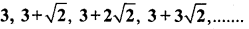(vi) 0.2, 0.22, 0.222, 0.2222,…
(vii) 0, – 4, – 8, – 12,…
(viii) $$-\frac{1}{2},-\frac{1}{2},-\frac{1}{2},-\frac{1}{2}$$ ,…………
(ix) 1, 3, 9, 27,………
(x) a, 2a, 3a, 4a,……….
(xi) a, a2, a3, a4,…
(xii)(xiii)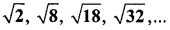(xiv) 12, 32,52, 72,………..
(xv) 12, 52, 72, 73,…….
i) 2, 4, 8, 16…………
d = a2 – a1= 4 – 2 = 2
d = a2 – a1 = 8 – 4 = 4
Here ‘d’ is not constant.
∴ the given List of numbers does not form an AP.

ii) $$2, \frac{5}{2}, 3, \frac{7}{2}$$,………..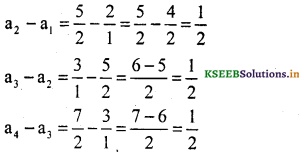∴ a2 – a1 = a3 – a2 = a4 – a3
∴ The given list of numbers from an A.P common difference = $$\frac{1}{2}$$
∴ Next three terms

∴ $$4, \frac{9}{2}, 5$$

iii) -1.2, -3.2. -5.2, -7.2
d = a2 – a1 = -3.2 – (-1.2) = -3.2+ 1.2
d = -2
d= a3 – a2 = -5.2 – (-3.2) = -5.2 + 3.2
d = -2
Here d is constant.
∴ This is an Arithmetic Progression.
Next three terms are:
-7.2 – 2 = -9.2
-9.2 – 2 = -11.2
-11.2 – 2 = -13.2
-11.2-2 = -13.2

iv) -10. -6. -2, 2,..,…
d = a2 – a1 = -6 – (- 10) = -6 + 10
d = 4
d = a3 – a2= -2 – (-6) = -2 + 6
d = 4
Here d is constant.
∴ Given set of numbers form an A.P.
Further three terms are
2 + 4 = 6
4 + 4 = 8
8 + 4 = 12v)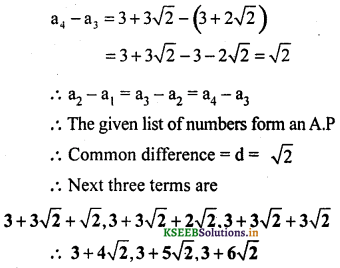vi) 0.2. 0.22. 0.222. 0.2222
d = a2 – a1 = 0.22 – 0.2
d = 0.02
d = a3 – a2 = 0.222 – 0.22
d = 0.002
Here d is not constant,
∴ Given set of numbers do not form an A.P.

vii) 0,-4, -8, -12, …………
a1 = 0, a2 = -4
d = a2 – a1 = -4 – 0
d = -4
d = a3 – a2 = -8 – (-4) = -8 + 4
d = -4
Here d is constant.
∴ Given a set of numbers form an AP.
Further three terms are:
-12 – 4 = -16
-16 – 4 = -20
-20 – 4 = -24
∴ Next three terms are: -16. -20, -24.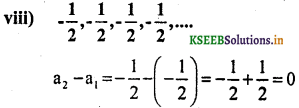Hence the given list of numbers form an A.P
Common difference = d = O
∴ Next three terms are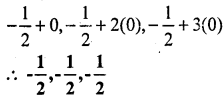ix) 1, 3, 9, 27
d = a2 – a1 = 3—1
d = 2
d = a3 – a2
d = 6
Here ‘d’ not is constant
∴ Given set of numbers do not form an A.P.

x) a, 2a, 3a, 4a
a1= a a2 = 2a
d = a2 – a1 = 2a-a
d = a
d = a3 – a2 = 3a-2a
d = a
Here d’ is constant.
∴ Given a set of numbers form an A.P.
Further three terms are :
4a + a = 5a
5a + a = 6a
6a + a = 7a
∴ Next three terms are: 5a, 6a, 7a.

xi) a, a2, a3, a4
a1 = a. a2 = a2
d = a2 – a1 = a2 – a
d= a(a – 1)
d = a3 – a2 = a3 – a2
d = a2 (a – 1)
Here ‘d is not constant.
∴ Given set of numbers do not form an A.P.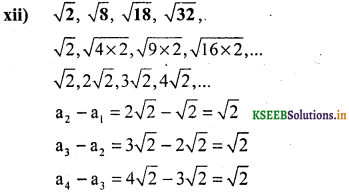∴ a – a1 ≠ a3 – a2 = a1 – a3
∴The given list of numbers form an A.P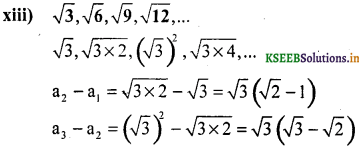a2 – a1 ≠ a3 – a2
∴ The given list of numbers does not form anA.P

xiv) 11,32,52, 72, …………
1, 9, 25, 49, ………….
a1 = 1, a2 = 9
d = a2 – a1 = 9 – 1
d = 8
d = a3 – a2 = 25 – 9
d = 16
Here ‘d’ is not constant,
∴ Given set of numbers do not form an A.P.

xv) 11, 52, 72, 73, ……….
1, 25. 49, 73, ……….
a1 = 1. a2 = 25, a3 = 49, a4 = 73
d = a2 – a1 = 25 – 1
d = 24
d = a3 – a2 = 49 – 25
d = 24
d = a4 – a3 = 73 – 49
d = 24
Here ‘d’ is constant.
∴ Given set of numbers form an A.P.
Succeeding three terms are:
73 + 24 = 97
97 + 24 = 121
121 + 24 = 145
∴ Next three terms are: 97, 121, 145.We hope the given KSEEB SSLC Class 10 Maths Solutions Chapter 1 Arithmetic Progressions Ex 1.1 will help you. If you have any query regarding Karnataka SSLC Class 10 Maths Solutions Chapter 1 Arithmetic Progressions Exercise 1.1, drop a comment below and we will get back to you at the earliest.